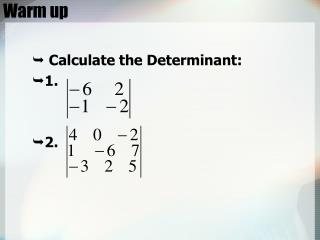DownloadDownload PresentationWarm up

# Warm up

Télécharger la présentation## Warm up

- - - - - - - - - - - - - - - - - - - - - - - - - - - E N D - - - - - - - - - - - - - - - - - - - - - - - - - - -
##### Presentation Transcript

1. Warm up • Calculate the Determinant: • 1. • 2.

2. Lesson 11-6 Cramer’s Rule Objective: To use Cramer’s Rule of Matrices to Solve Systems of Equations

3. Cramer’s Rule - 2 x 2 • Cramer’s Rule relies on determinants. • Consider the system below with variables x and y:

4. Cramer’s Rule - 2 x 2 • The formula for the values of x and y are shown below. The numbers inside the determinants are the coefficients and constants from the equations. Replace the first column with the constant matrix Replace the second column with the constant matrix Det. of the Coefficient matrix

5. Use Cramer’s rule to solve this system: 9x + 4y = - 6 3x - 5y = - 21 9 4 3 –5 Use Cramer’s rule for a 2 X 2 system EXAMPLE SOLUTION STEP 1 Evaluate the determinant of the coefficient matrix. = –45 – 12 = –57

6. STEP 2 Apply Cramer’s rule because the determinant is not 0. –6 4 –5 30 – (–84) 114 –21 –2 = = x = = –57 –57 –57 9 –6 3 –21 –171 –189 – (–18) 3 y = = = = –57 –57 –57 ANSWER The solution is ( -2, 3). Use Cramer’s rule for a 2 X 2 system

7. Cramer’s Rule • Example: Solve the system:3x - 2y = 10 4x + y = 6 The solution is (2, -2)

8. Cramer’s Rule • Not all systems have a definite solution. If the determinant of the coefficient matrix is zero, a solution cannot be found using Cramer’s Rule due to division by zero. • When the solution cannot be determined, one of two conditions exists: • The planes graphed by each equation are parallel and there are no solutions. • The three planes share one line (like three pages of a book share the same spine) or represent the same plane, in which case there are infinite solutions.

9. Cramer’s Rule • Example:Solve the system3x - 2y + z = 9x + 2y - 2z = -5x + y - 4z = -2

10. Cramer’s Rule • Example, continued: 3x - 2y + z = 9 x + 2y - 2z = -5x + y - 4z = -2 The solution is (1, -3, 0)

11. 2. 4x + 7y = 2 –3x – 2y = 28 ANSWER ANSWER ANSWER (–1, 3). (4, –2). (2, –1, 4). PRACTICE Use Cramer’s rule to solve the linear system. 1. 3x –4y = –15 3. 3x –4y + 2z = 18 2x + 5y = 13 4x + y – 5z = –13 2x –3y + z = 11